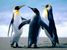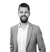# App Development

Announcements
An issue has been identified on Qlik Cloud hub, please visit our Status Update Page for details: GET THE LATEST
cancel
Showing results for
Did you mean:Contributor III

## Calculate Column

What I would like to do if possible is to get the sum total minus value and the sum total possitive value from a column in the table

the expression for the field is

=num((aggr(sum(TOTQTY),PART_NUMBER) -aggr(sum({<[HM000_WAREHOUSE]={1}>}[HM000_MOHTQ]),PART_NUMBER,[LOCATIONS-SYNONM],Planner))*[Standard Cost],'£#,##0.00;-£#,##0.00')

any help would be  greatly appreciated

1 Solution

Accepted SolutionsPartner - Master

Hi Eaun,

The reason why this is not working, is because you are creating two arrays and subtracting them from each other. Try to put a sum around the aggrs:

``=num(Sum(aggr(sum(TOTQTY),PART_NUMBER)) - Sum(aggr(sum({<[HM000_WAREHOUSE]={1}>}[HM000_MOHTQ]),PART_NUMBER,[LOCATIONS-SYNONM],Planner))*[Standard Cost],'£#,##0.00;-£#,##0.00')``

Jordy

Climber

Work smarter, not harder
2 RepliesPartner - Master

Hi Eaun,

The reason why this is not working, is because you are creating two arrays and subtracting them from each other. Try to put a sum around the aggrs:

``=num(Sum(aggr(sum(TOTQTY),PART_NUMBER)) - Sum(aggr(sum({<[HM000_WAREHOUSE]={1}>}[HM000_MOHTQ]),PART_NUMBER,[LOCATIONS-SYNONM],Planner))*[Standard Cost],'£#,##0.00;-£#,##0.00')``

Jordy

Climber

Work smarter, not harderContributor III
Author

Thanks that workedTags
Community Browser